# Borsuk-Ulam Theorem (The global version)

$\ \ \ \ \ $/extract_itex] In mathematics, the Borsuk-Ulam theorem states that every continuous function from an $n$-sphere into Euclidean $n$-space maps some pair of antipodal points to the same point. A pair of points on a sphere are called antipodal if they are in exactly opposite directions from the sphere's center or that if they are situated on diametrical end points of any diameter of the sphere. Formally, if $\displaystyle f:S^{n}\to \mathbb {R} ^{n}$ is continuous then there exists an $\displaystyle x\in S^{n}$ such that: $\displaystyle f(-x)=f(x)$. Here $S^n$ represents the $n^{th}$-sphere and $\mathbb R$ denotes the set of real numbers.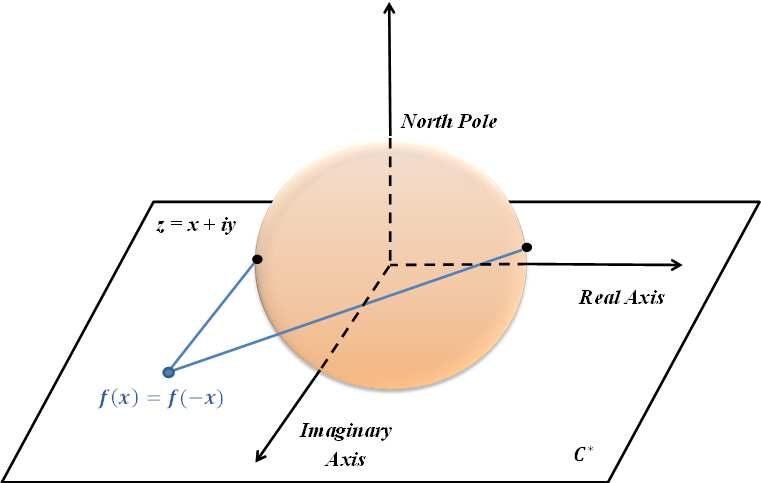$\ \ \ \ \ \$ A spin-off to this mathematical theorem states that at a given instant, there exists at least one such pair of antipodal points on the earth which have the same temperature and pressure. $\ \ \ \ \ \$ At first glance, this statement seems hard to prove, but in fact it has a very simple approach which we will be going through in this note. Statement At a given instant there exists at least one pair of antipodal points on the earth which have the same temperature and same pressure. Proof Take two thermometers any two random antipodal points $A$ and $B$ on the surface of the Earth such that at this particular instant their temperature readings are different. Without loss of generality, assume that the temperature at point $A$ is higher than that of $B$. Now swap the positions of the thermometers in such a manner that they always remain antipodal at any point in their paths.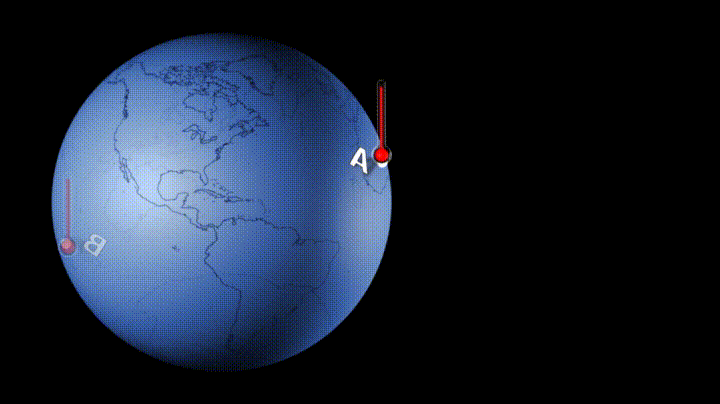In the process of swapping, observe that there is at least one antipodal point where the temperature readings of both the thermometers were equal.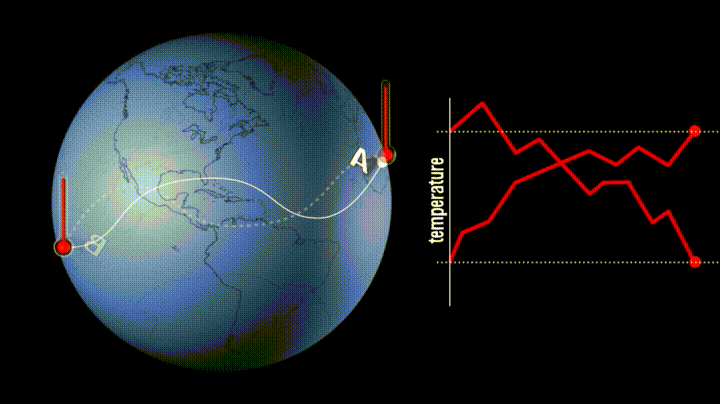Thus, we have proved the existence of two antipodal points on the Earth at any instant whose temperatures are equal. $\\ \\$ Now, repeating the same process with infinitely many sets of antipodal points $A$ and $B$ gives us a band of antipodal points on the surface of the Earth. There will be no existence of gaps between any two points on this band due to the fact that if there exists a gap, then we would be able to swap the thermometers antipodally between $A$ and $B$ without ever encountering an intersection where the temperature readings meet, which is not possible.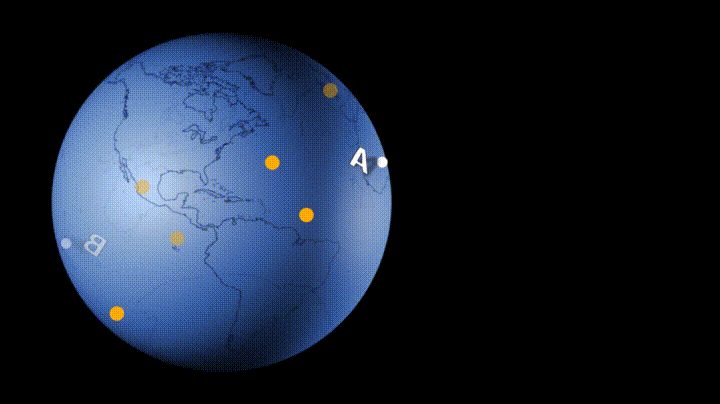Now take any two random antipodal points on this band and measure the atmospheric pressure at each point using a barometer. Again, swap the barometers between these two points but in the process of swapping, only move on the band of antipodally isothermic points. Again, in the process of swapping we will encounter at least one point where the pressure graphs intersect and at this point the pressure readings on both the barometers will be the same.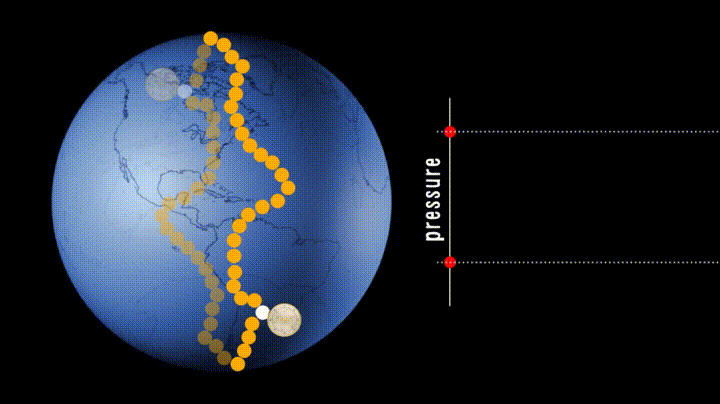Since, we were already moving along antipodally isothermic points on the earth, this pair of antipodal points will have both temperature as well as pressure equal. Thus we have also shown the existence of at least one pair of antipodal points on Earth, where at a particular instant, both the temperature and pressure readings will be respectively equal to one another. $\ \ \ \ \ \ \ \ \ \ \ \ \ \ \ \ \ \ \ \ \ \ \ \ \ \ \ \ \ \ \ \ \ \ \ \ \ \ \ \ \ \ \ \ \ \ \ \ \ \ \ \ \ \ \ \ \ \ \ \ \ \ \ \ \ \ \ \ \ \ \ \ \ \ \ \ \ \ \ \ \ \ \ \ \ \ \ \ \ \ \ \ \ \ \ \ \ \ \ \ \ \ \ \ \ \ \ \ \ \ \ \ \ \ \ \ \ \ \ \ \ \ \ \ \ \ \ \ \ \ \ \ \ \ \ \ \ \ \ \ \ \ \ \ \ \ \ \ \ \ \ \ \ \ \ \ \ \ \ \ \ \ \ \ \ \ \ \ \ \ \ \ \ \ \ \ \ \ \ \ \ \ \ \ \ \ \ \ \ \ \text{Q.E.D.}$ However, this approach is only applicable in theory and cannot be shown practically as both the temperature and pressure at a given point on Earth keep on changing continuously at variable rates. Hope you enjoyed reading this. $\ \ \ \ \ \ \ \ \ \ \ \ \ \ \ \ \ \ \ \ \ \ \ \ \ \ \ \ \ \ \ \ \ \ \ \ \ \ \ \ \ \ \ \ \ \ \ \ \ \ \ \ \ \ \ \ \ \ \ \ \ \ \ \ \ \ \ \ \ \ \ \ \ \ \ \ \ \ \ \ \ \ \ \ \ \ \ \ \ \ \ \ \ \ \ \ \ \ \ \ \ \ \ \ \ \ \ \ \ \ \ \ \ \ \ \ \ \ \ \ \ \ \ \ \ \ \ \ \ \ \ \ \ \ \ \ \ \ \ \ \ \ \ \ \ \ \ \ \ \ \ \ \ \ \ \ \ \ \ \ \ \ \ \ \ \ \ \ \ \ \ \ \ \ \ \ \ \ \ \ \ \ \ \ \ \ \ \ \ \ Thank \ you$ ### Source: Vsauce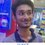Note by Tapas Mazumdar 4 years, 9 months ago This discussion board is a place to discuss our Daily Challenges and the math and science related to those challenges. Explanations are more than just a solution — they should explain the steps and thinking strategies that you used to obtain the solution. Comments should further the discussion of math and science. When posting on Brilliant: • Use the emojis to react to an explanation, whether you're congratulating a job well done , or just really confused . • Ask specific questions about the challenge or the steps in somebody's explanation. Well-posed questions can add a lot to the discussion, but posting "I don't understand!" doesn't help anyone. • Try to contribute something new to the discussion, whether it is an extension, generalization or other idea related to the challenge. • Stay on topic — we're all here to learn more about math and science, not to hear about your favorite get-rich-quick scheme or current world events. MarkdownAppears as *italics* or _italics_ italics **bold** or __bold__ bold - bulleted- list • bulleted • list 1. numbered2. list 1. numbered 2. list Note: you must add a full line of space before and after lists for them to show up correctly paragraph 1paragraph 2 paragraph 1 paragraph 2 [example link](https://brilliant.org)example link > This is a quote This is a quote  # I indented these lines # 4 spaces, and now they show # up as a code block. print "hello world" # I indented these lines # 4 spaces, and now they show # up as a code block. print "hello world" MathAppears as Remember to wrap math in $$ ... $$ or \[ ... $ to ensure proper formatting.
2 \times 3 $2 \times 3$
2^{34} $2^{34}$
a_{i-1} $a_{i-1}$
\frac{2}{3} $\frac{2}{3}$
\sqrt{2} $\sqrt{2}$
\sum_{i=1}^3 $\sum_{i=1}^3$
\sin \theta $\sin \theta$
\boxed{123} $\boxed{123}$

Sort by:

Great. This would make a good start to the Borsuk Ulam Theorem wiki. Can you help us get started?

Staff - 4 years, 9 months ago